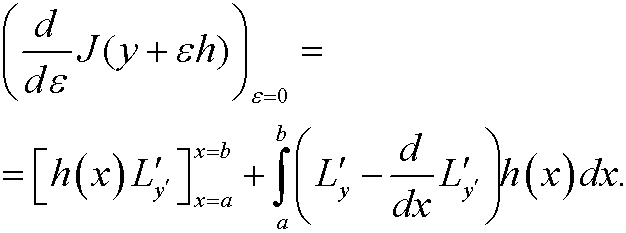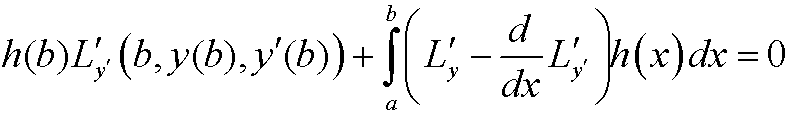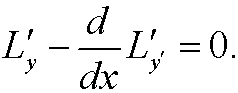# 9. Natural boundary conditions.

Assume that we instead had the boundary conditions y(a)=y0, y(b)undetermined, in theorem 1. Such a problem is called a free-boundary value problem. Now choose the function h such thatwithout posing any condition on h in the point x=b. By imitating the proof of the original theorem we see thatSince h(a)=0 a necessery condition for y  to be extremal isfor everySince this condition holds for all h above, it in particular holds for those hthat fulfills h(b)=0. This implies thatand by the lemma in the previous part, we realize that y  must fulfill the Euler equationIf we again let h(b) be arbitrary and return to the necessary condition above we see that when we insert the Euler equation that we must have(for all choices of h(b) ). Since this is independent of h(b) we must haveThis is the natural boundary condition.

Example 13: Find the differential equation and the boundary conditions for the extremal to the functionalwhen y(0)=0, y(1) undetermined.

<>

Solution:The Euler equation is thenthat is,The natural boundary condition in x=1 isThis means that the boundary conditions are